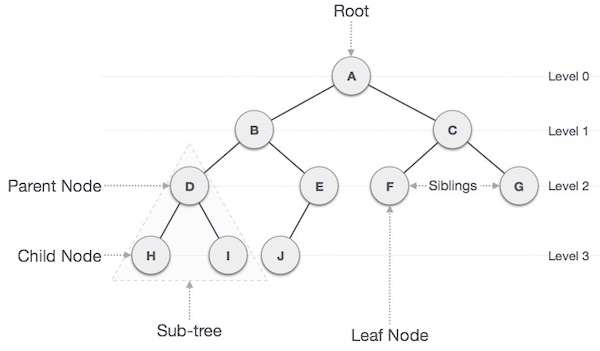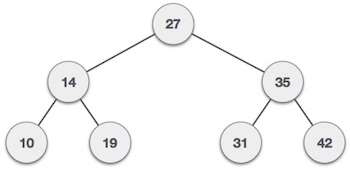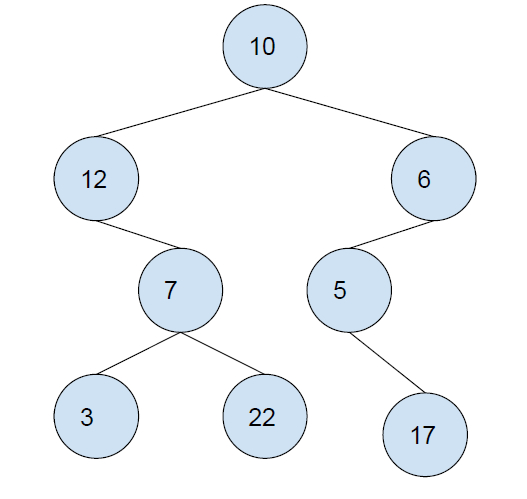# Maximum sub-tree sum in a Binary Tree such that the sub-tree is also a BST in C++ program

In this problem, we are given a binary tree BT. Our task is to create a program to find the Maximum sub−tree sum in a Binary Tree such that the sub−tree is also a BST.

Binary Tree has a special condition that each node can have a maximum of two children.Binary Search Tree is a tree in which all the nodes follow the below−mentioned properties

• The value of the key of the left sub-tree is less than the value of its parent (root) node's key.

• The value of the key of the right subtree is greater than or equal to the value of its parent (root) node's key.Let’s take an example to understand the problem,

## Input## Output

32

## Explanation

Here, we have two subtrees that are BST. Their sum is,

7 + 3 + 22 = 32
6 + 5 + 17 = 28
Maximum = 32.

## Solution Approach

A simple solution to the problem is by traversing the tree data structure and then checking at each node if it’s child nodes can form a binary Search tree or not. If we find the sum of all nodes contributing to the BST and then return the maximum of all BSTSum’s found.

## Example

Program to illustrate the working of our solution,

Live Demo

#include <bits/stdc++.h>
using namespace std;
int findMax(int a, int b){
if(a > b)
return a;
return b;
}
int findMin(int a, int b){
if(a > b)
return b;
return a;
}
struct Node {
struct Node* left;
struct Node* right;
int data;
Node(int data){
this−>data = data;
this−>left = NULL;
this−>right = NULL;
}
};
struct treeVal{
int maxVal;
int minVal;
bool isBST;
int sum;
int currMax;
};
treeVal CalcBSTSumTill(struct Node* root, int& maxsum){
if (root == NULL)
return { −10000, 10000, true, 0, 0 };
if (root−>left == NULL && root−>right == NULL) {
maxsum = findMax(maxsum, root−>data);
return { root−>data, root−>data, true, root−>data, maxsum };
}
treeVal LeftSTree = CalcBSTSumTill(root−>left, maxsum);
treeVal RightSTree = CalcBSTSumTill(root−>right, maxsum);
treeVal currTRee;
if (LeftSTree.isBST && RightSTree.isBST && LeftSTree.maxVal <
root−>data && RightSTree.minVal > root−>data) {
currTRee.maxVal = findMax(root−>data,
findMax(LeftSTree.maxVal, RightSTree.maxVal));
currTRee.minVal = findMin(root−>data,
findMin(LeftSTree.minVal, RightSTree.minVal));
maxsum = findMax(maxsum, RightSTree.sum + root−>data +
LeftSTree.sum);
currTRee.sum = RightSTree.sum + root−>data +
LeftSTree.sum;
currTRee.currMax = maxsum;
currTRee.isBST = true;
return currTRee;
}
currTRee.isBST = false;
currTRee.currMax = maxsum;
currTRee.sum = RightSTree.sum + root−>data + LeftSTree.sum;
return currTRee;
}
int CalcMaxSumBST(struct Node* root){
int maxsum = −10000;
return CalcBSTSumTill(root, maxsum).currMax;
}
int main(){
struct Node* root = new Node(10);
root−>left = new Node(12);
root−>left−>right = new Node(7);
root−>left−>right−>left = new Node(3);
root−>left−>right−>right = new Node(22);
root−>right = new Node(6);
root−>right−>left = new Node(5);
root−>right−>left−>right = new Node(17);
cout<<"The maximum sub−tree sum in a Binary Tree such that the
sub−tree is also a BST is "<<CalcMaxSumBST(root);
return 0;
}

## Output

The maximum sub−tree sum in a Binary Tree such that the sub−tree is also a BST is 32